# Nonparametric Regression

Given the usual conditional expectation function

$E[y_i | \mathbf{x_i} = \mathbf{x}] = m(x)$

we can estimate $$m(x)$$ as a traditional linear model or nonparametrically. One approach to nonparametric estimation is to use a binned means estimator. This takes the average $$y$$ for some values of $$x_i$$ close to $$x$$ (assuming $$x_i$$ is continuous). In other words, take the average $$y_i$$ for all values $$x_i$$ where $$|x_i - x| \leq h$$ for some small $$h$$. Here $$h$$ is the bandwidth.

### Binned means estimator

$\begin{equation} \widehat{m}(x) = \frac{\sum_{i=1}^n \mathbb{1}(|x_i - x| \leq h)y_i}{\sum_{i=1}^n \mathbb{1}(|x_i - x|\leq h)} \end{equation}$

This gives us a step-function because the indicator function makes the weights discontinuous. Alternatively, we can replace the indicator function with a kernel function:

### Kernel regression estimator

$\begin{equation} \widehat{m}_k(x) = \frac{\sum_{i=1}^n K\left(\frac{x_i - x}{h}\right)y_i}{\sum_{i=1}^n K\left(\frac{x_i - x}{h}\right)} \end{equation}$

Some commonly used kernels:

Gaussian Kernel: $K(u) = \frac{1}{\sqrt{2 \pi}} exp \left (-\frac{u^2}{2}\right)$

Epanechnikov Kernel: $K(u) = \left\{ \begin{array}{ll} \frac{3}{4\sqrt{5}}\left(1 - \frac{u^2}{5}\right) & \mbox{if |u| < \sqrt{5}}\\ 0 & \mbox{otherwise}\end{array} \right.$

### Local Linear approximation

Let’s start with simulating some data using the simstudy packge:

library(tidyverse)
## simulate data using simstudy package
library(simstudy)
set.seed(234)
df <- genData(100, defData(varname = "x", formula = "20;60", dist = 'uniform'))
theta1 = c(0.1, 0.8, 0.6, 0.4, 0.6, 0.9, 0.9)
knots <- c(0.25, 0.5, 0.75)
df <- genSpline(dt = df, newvar = "y",
predictor = "x",
theta = theta1,
knots = knots,
degree = 3,
newrange = "90;160",
noise.var = 64)

Here is a scatterplot with dashed vertical lines showing the knots used to generate the data (quantiles).

## scatter plot of the data
ggplot(data = df, aes(x = x, y = y)) +
geom_point() +
geom_vline(xintercept = quantile(df$x, knots), linetype = 'dashed') + theme_bw()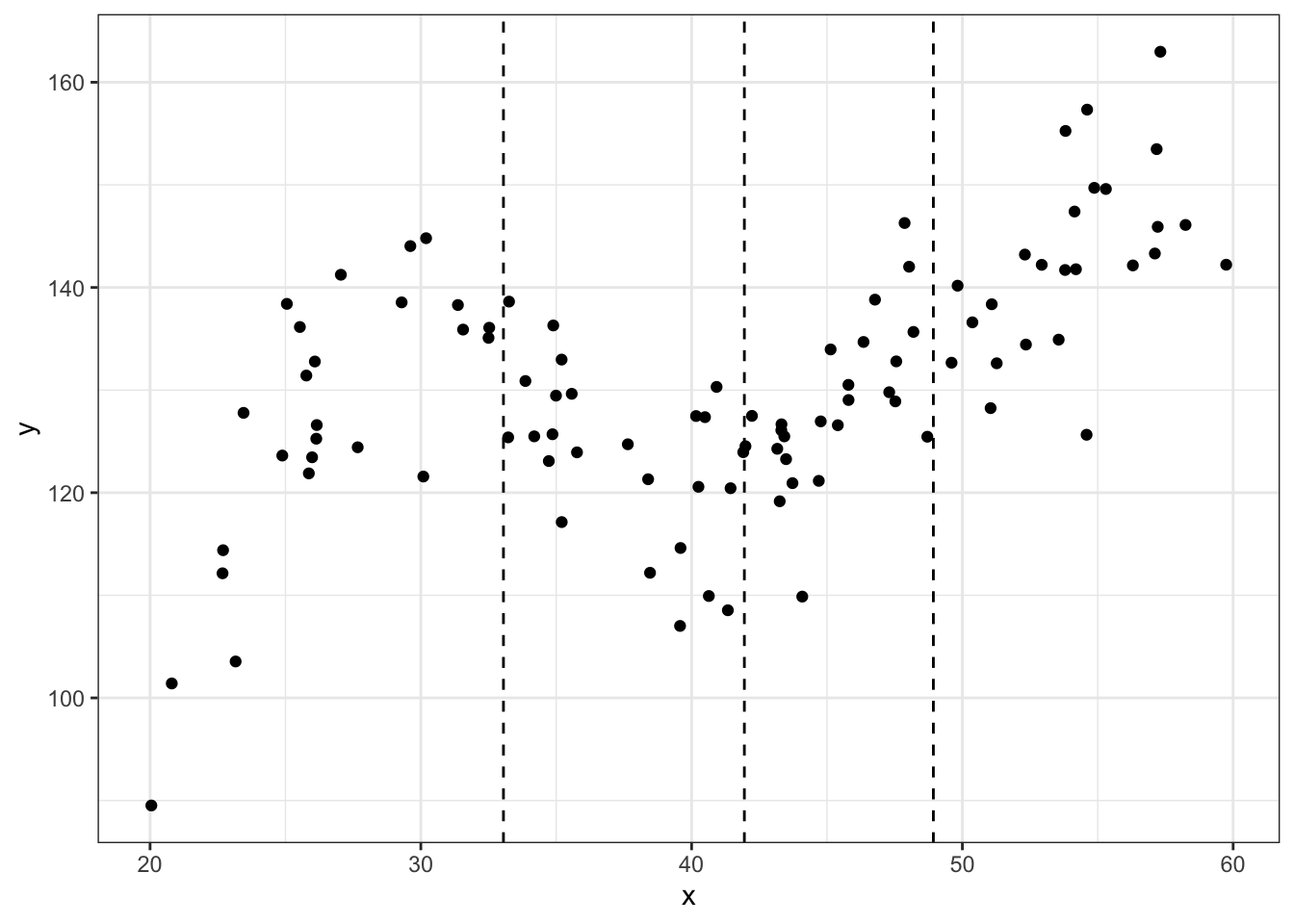#using locpoly library(KernSmooth) ## KernSmooth 2.23 loaded ## Copyright M. P. Wand 1997-2009 m_ll <- data.frame(locpoly(df$x, df$y, degree = 1, bandwidth = 3)) # degree = 1 for locally linear ggplot(data = df, aes(x = x, y = y)) + geom_point() + geom_vline(xintercept = quantile(df$x, knots), linetype = 'dashed') +
geom_line(data = m_ll,aes(x = x, y = y),color = "blue") +
theme_bw()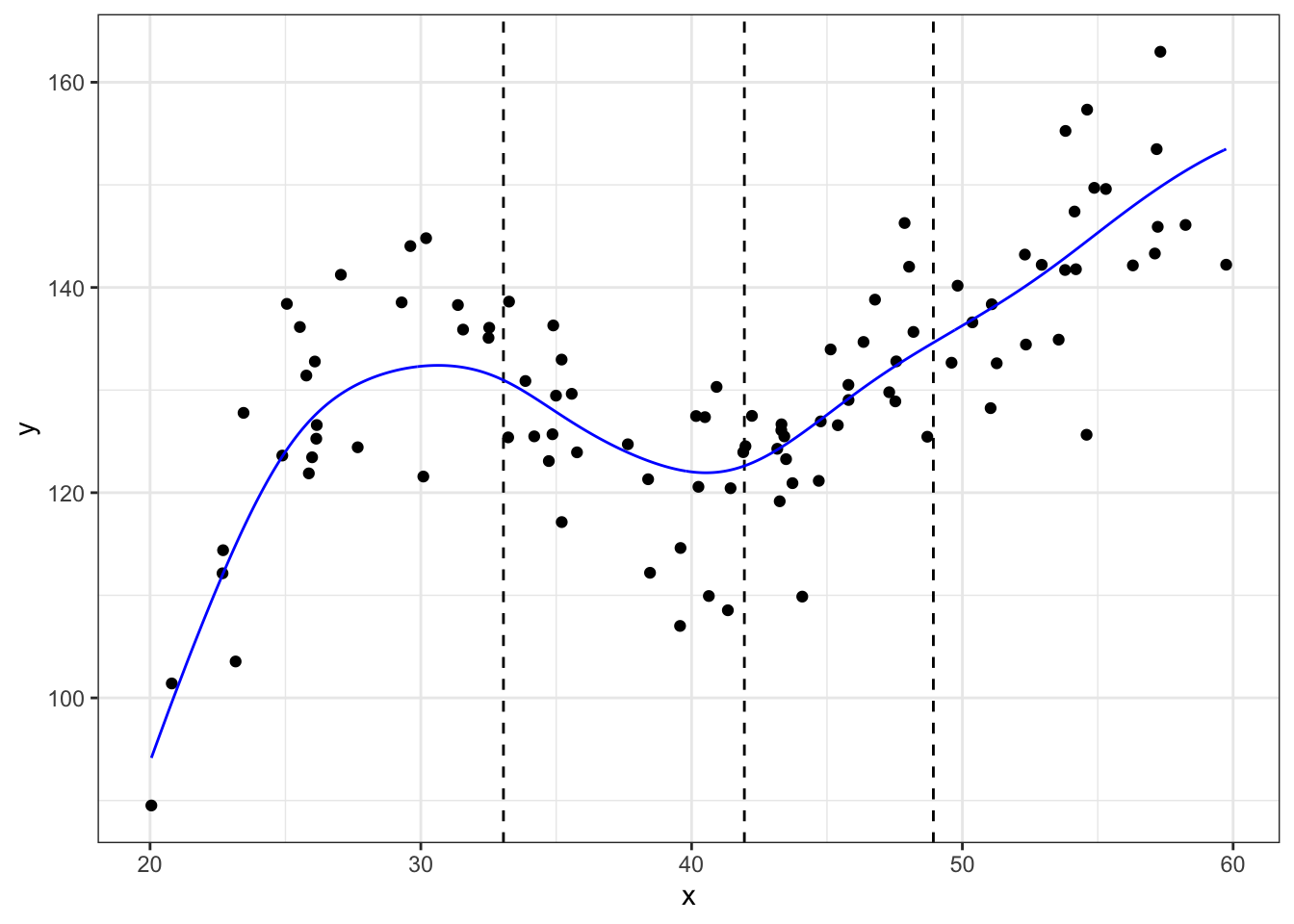tricube kernal $K(u) = \left\{ \begin{array}{ll} (1-|u|^3)^3 & \mbox{for |u| < 1}\\ 0 & \mbox{for |u|\geq 1 }\end{array} \right.$

kernel.function <- function(u){
tmp <- rep(NA,length(u))
for(i in 1:length(u)){
if(abs(u[i])<1){
k <- (1 - abs(u[i])^3)^3
}else if(abs(u[i])>=1){
k <- 0
}
tmp[i] <- k
}
return(tmp)
}

Decide on evaluation points $$x_0$$. Our data is (roughly) from 20 to 60 so let’s use that to define our evaluation points and we will use increments of 20.

# evaluation points
x0 <- seq(25,55,10)
# bandwidth
h <- 10

We have 3 evaluation points, let’s do a loop to calculate the scaled distance from each value of $$x$$ to $$x_0$$.

# empty container
distances <- matrix(NA, nrow = length(df$x), ncol = 4) colnames(distances) <- x0 for(i in 1:length(x0)){ # calc distance and put in column i distances[,i] <- df$x - x0[i]
# divide by sum of bandwidth (this does scaling)
# want scaled so can use as weights
#distances[,i] <- distances[,i]/sum(distances[,i])
}

Now apply our kernel function to estimate the weights

distances <- data.frame(distances)
weights <- map_df(distances, kernel.function)

Estimate predicted values at the evaluation points using our weights

# eval point 20
W_25 <- diag(weights$X25) X <- as.matrix(cbind(rep(1,100), df$x)) #create X matrix with intercept
Y <- as.matrix(df$y) B_WLS_25 <- solve(t(X)%*%W_25%*%X)%*%(t(X)%*%W_25%*%Y) # eval point 40 W_45 <- diag(weights$X45)
B_WLS_45 <- solve(t(X)%*%W_45%*%X)%*%(t(X)%*%W_45%*%Y)
# eval point 60
W_55 <- diag(weights$X55) B_WLS_55 <- solve(t(X)%*%W_55%*%X)%*%(t(X)%*%W_55%*%Y) # predicted values yhat <- data.frame(y25 = X%*%B_WLS_25, y45 = X%*%B_WLS_45, y55 = X%*%B_WLS_55) yhat <- pivot_longer(yhat, names_to = "group", names_prefix = "y", values_to="yhat",cols = c(1:3)) df_big <- bind_cols(bind_rows(df,df,df),yhat) #first eval point ggplot(data = df_big %>% filter(group==25), aes(x = x, y = y)) + geom_point() + geom_point(aes(x = x, y = yhat),color="red") + theme_bw()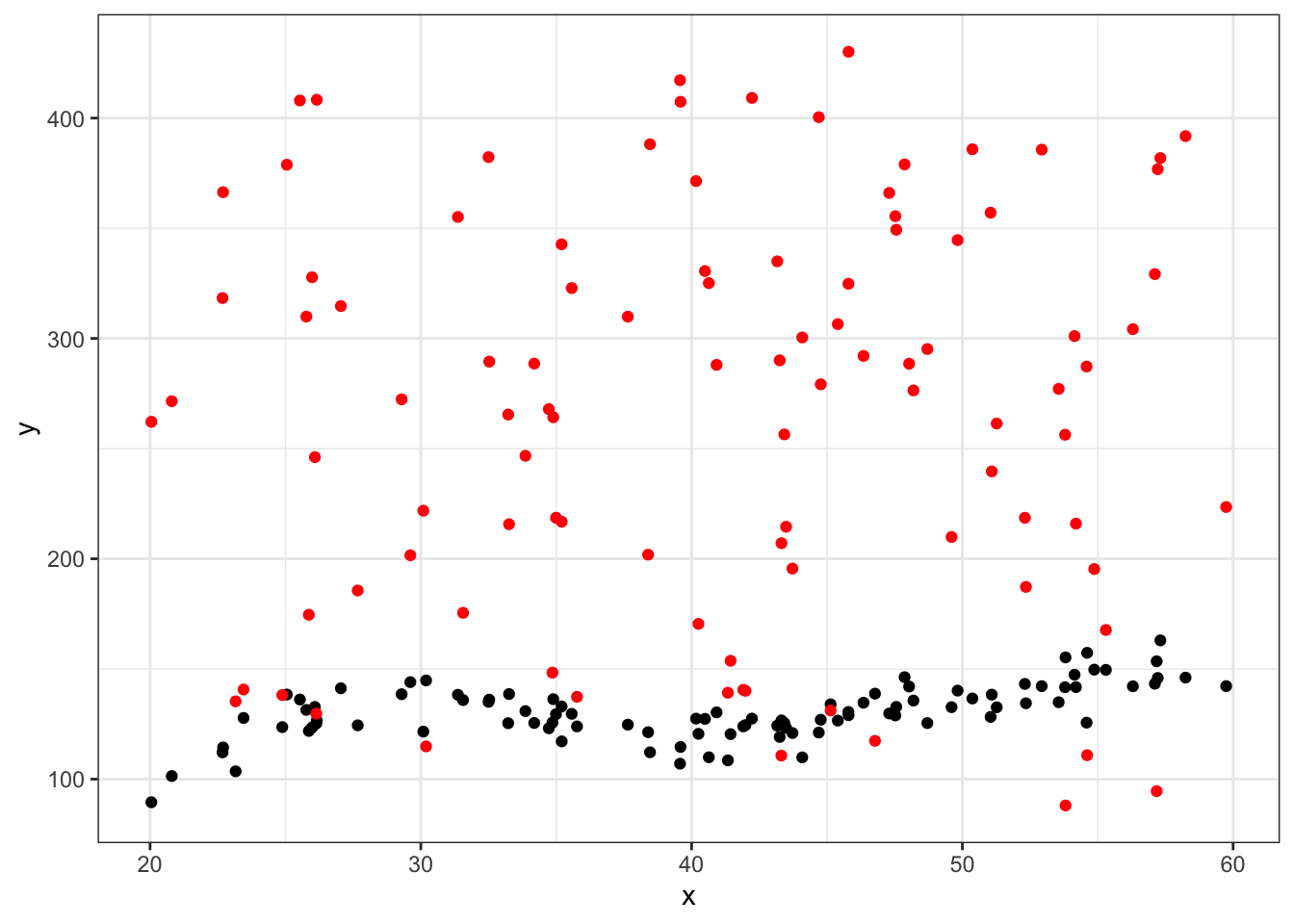ggplot(data = df_big %>% filter(group==45), aes(x = x, y = y)) + geom_point() + geom_point(aes(x = x, y = yhat), color="green") + theme_bw()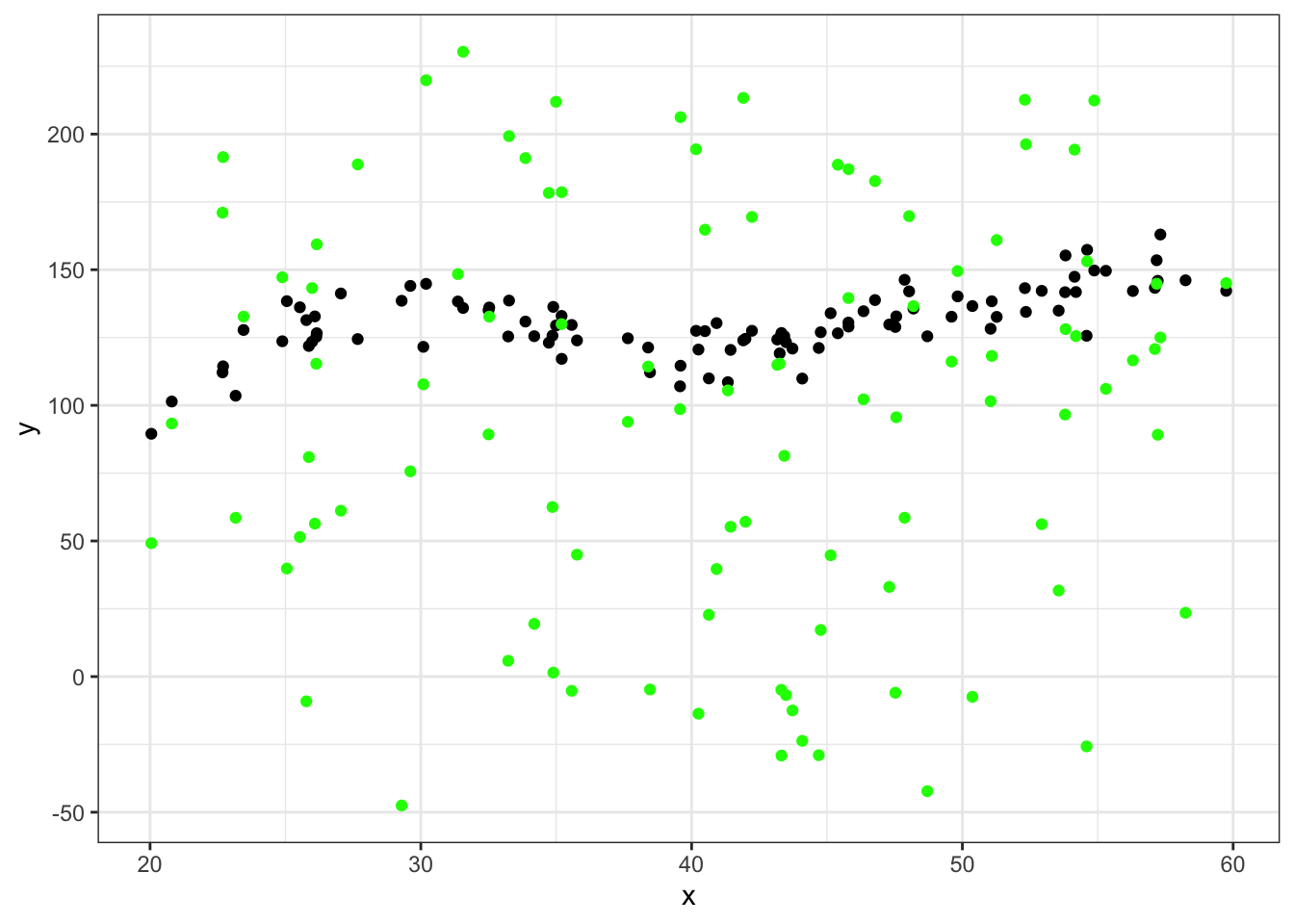ggplot(data = df_big %>% filter(group==60), aes(x = x, y = y)) + geom_point() + geom_point(aes(x = x, y = yhat), color="blue") + theme_bw()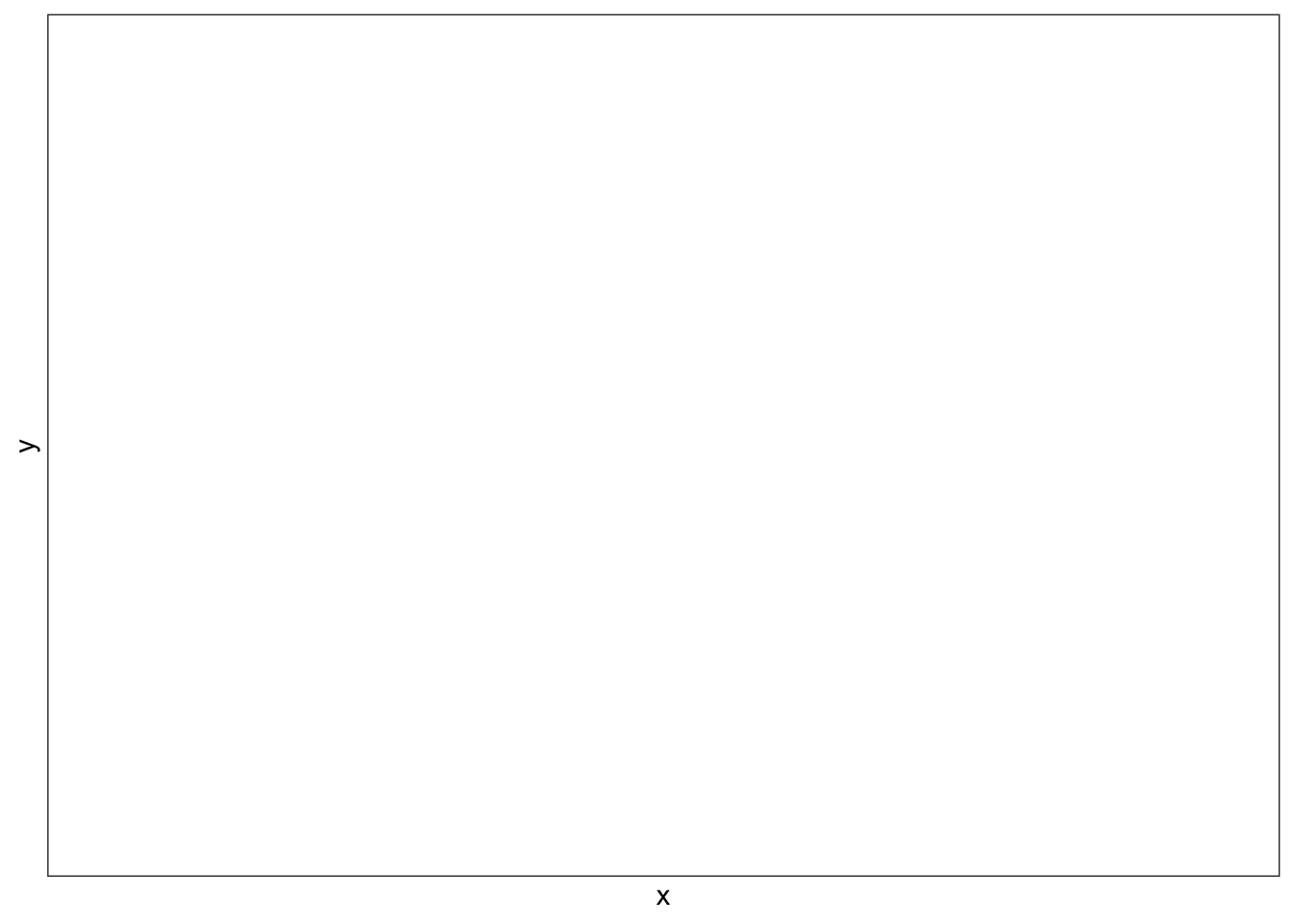ggplot(data = df_big, aes(x = x, y = y)) + geom_point() + geom_point(aes(x = x, y = yhat, color=group)) + theme_bw()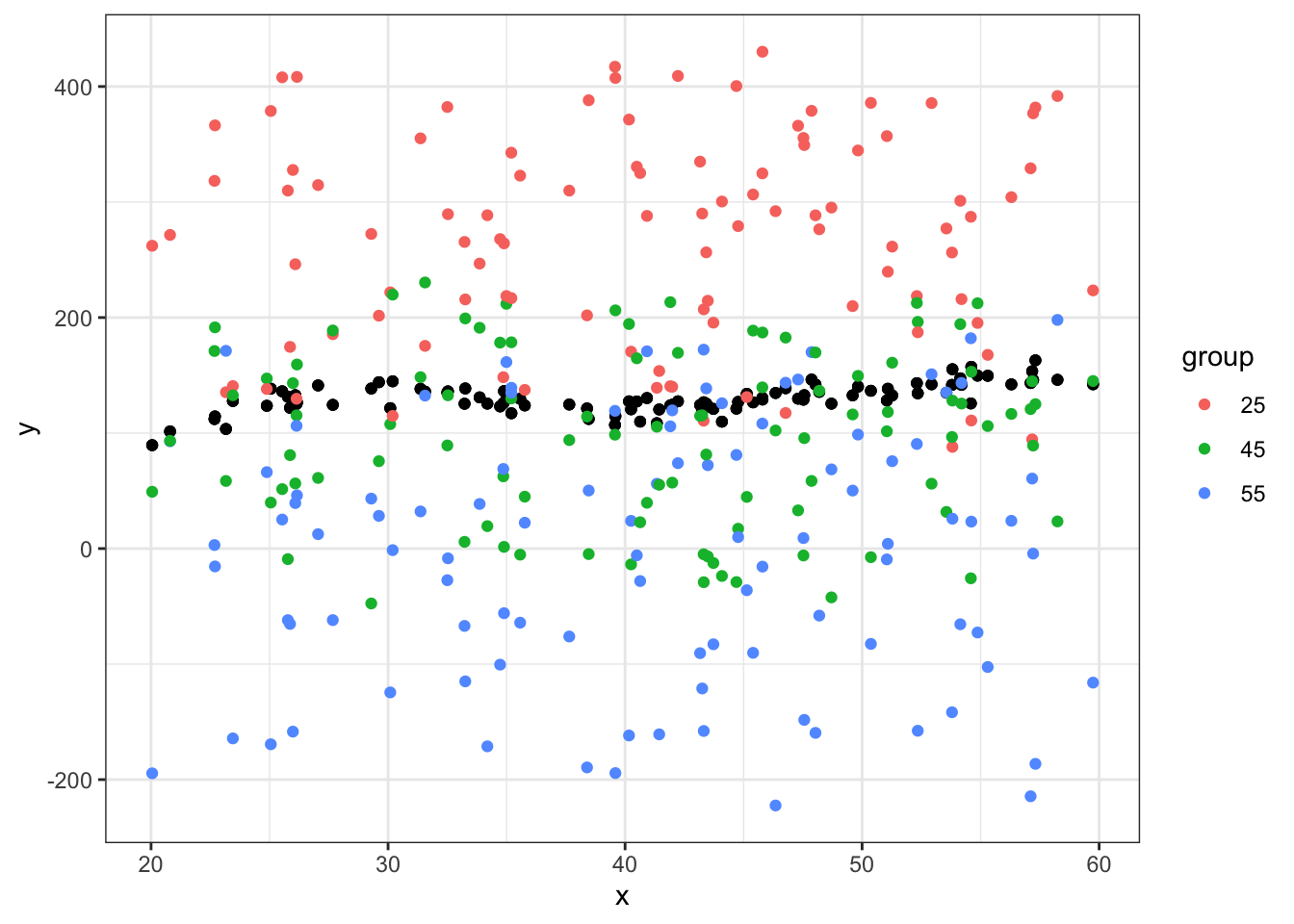Switching gears – let’s do a quick example on how to demean variables using dplyr. We will use the built-in mtcars dataset. First, let’s demean only one column, the mpg column and make a new variable using mutate(): df <- mtcars %>% mutate(mpg_demean = mpg - mean(mpg)) You have to assign this to an object, in this case I’ve stored it as an entirely new data.frame df. I can now use this new variable: head(df$mpg_demean)
##   0.909375  0.909375  2.709375  1.309375 -1.390625 -1.990625

Here, the demeaned variable is calculated but not stored because I have not assigned this operation to anything. If I try to call the variable mpg_demean later I will get an error because it does not exist.

mtcars %>%
mutate(mpg_demean2 = mpg - mean(mpg)) %>%
head()
##    mpg cyl disp  hp drat    wt  qsec vs am gear carb mpg_demean2
## 1 21.0   6  160 110 3.90 2.620 16.46  0  1    4    4    0.909375
## 2 21.0   6  160 110 3.90 2.875 17.02  0  1    4    4    0.909375
## 3 22.8   4  108  93 3.85 2.320 18.61  1  1    4    1    2.709375
## 4 21.4   6  258 110 3.08 3.215 19.44  1  0    3    1    1.309375
## 5 18.7   8  360 175 3.15 3.440 17.02  0  0    3    2   -1.390625
## 6 18.1   6  225 105 2.76 3.460 20.22  1  0    3    1   -1.990625
head(df$mpg_demean2) ## NULL If I want to de-mean multiple variables at once use mutate_each. Here I am selecting two columns, vs and am, then using mutate_all to demean them both then I’m joining them back to the original data. df <- df %>% select(vs, am) %>% mutate_all(list(~. - mean(.))) %>% bind_cols(df,.) ## New names: ## * vs -> vs...8 ## * am -> am...9 ## * vs -> vs...13 ## * am -> am...14 head(df) ## mpg cyl disp hp drat wt qsec vs...8 am...9 gear carb mpg_demean vs...13 ## 1 21.0 6 160 110 3.90 2.620 16.46 0 1 4 4 0.909375 -0.4375 ## 2 21.0 6 160 110 3.90 2.875 17.02 0 1 4 4 0.909375 -0.4375 ## 3 22.8 4 108 93 3.85 2.320 18.61 1 1 4 1 2.709375 0.5625 ## 4 21.4 6 258 110 3.08 3.215 19.44 1 0 3 1 1.309375 0.5625 ## 5 18.7 8 360 175 3.15 3.440 17.02 0 0 3 2 -1.390625 -0.4375 ## 6 18.1 6 225 105 2.76 3.460 20.22 1 0 3 1 -1.990625 0.5625 ## am...14 ## 1 0.59375 ## 2 0.59375 ## 3 0.59375 ## 4 -0.40625 ## 5 -0.40625 ## 6 -0.40625 Using base R you could do something like this: df$wt_demean <- df$wt - mean(df$wt)
head(df)
##    mpg cyl disp  hp drat    wt  qsec vs...8 am...9 gear carb mpg_demean vs...13
## 1 21.0   6  160 110 3.90 2.620 16.46      0      1    4    4   0.909375 -0.4375
## 2 21.0   6  160 110 3.90 2.875 17.02      0      1    4    4   0.909375 -0.4375
## 3 22.8   4  108  93 3.85 2.320 18.61      1      1    4    1   2.709375  0.5625
## 4 21.4   6  258 110 3.08 3.215 19.44      1      0    3    1   1.309375  0.5625
## 5 18.7   8  360 175 3.15 3.440 17.02      0      0    3    2  -1.390625 -0.4375
## 6 18.1   6  225 105 2.76 3.460 20.22      1      0    3    1  -1.990625  0.5625
##    am...14 wt_demean
## 1  0.59375  -0.59725
## 2  0.59375  -0.34225
## 3  0.59375  -0.89725
## 4 -0.40625  -0.00225
## 5 -0.40625   0.22275
## 6 -0.40625   0.24275

If I want to demean by groups. Here I will use the cylinder variable to group with.

df <- df %>%
group_by(cyl) %>%
mutate(mpg_mean_cyl = mpg - mean(mpg))
head(df[,c("mpg","cyl","mpg_mean_cyl")])
## # A tibble: 6 x 3
## # Groups:   cyl 
##     mpg   cyl mpg_mean_cyl
##   <dbl> <dbl>        <dbl>
## 1  21       6         1.26
## 2  21       6         1.26
## 3  22.8     4        -3.86
## 4  21.4     6         1.66
## 5  18.7     8         3.6
## 6  18.1     6        -1.64

Here is an example of some code that runs but may not be doing what we want. What are some differences in this code?

df %>%
group_by(cyl) %>%
mutate(new_mpg = mean(mpg, na.rm=TRUE)) %>%
select(mpg,new_mpg)
## Adding missing grouping variables: cyl
## # A tibble: 32 x 3
## # Groups:   cyl 
##      cyl   mpg new_mpg
##    <dbl> <dbl>   <dbl>
##  1     6  21      19.7
##  2     6  21      19.7
##  3     4  22.8    26.7
##  4     6  21.4    19.7
##  5     8  18.7    15.1
##  6     6  18.1    19.7
##  7     8  14.3    15.1
##  8     4  24.4    26.7
##  9     4  22.8    26.7
## 10     6  19.2    19.7
## # … with 22 more rows

## F-test

We use the F-test to look at multiple hypotheses at once.

fit <- lm(mpg ~ cyl + vs + am + gear + carb, data = mtcars)
summary(fit)
##
## Call:
## lm(formula = mpg ~ cyl + vs + am + gear + carb, data = mtcars)
##
## Residuals:
##     Min      1Q  Median      3Q     Max
## -5.5403 -1.1582  0.2528  1.2787  5.5597
##
## Coefficients:
##             Estimate Std. Error t value Pr(>|t|)
## (Intercept)  25.1591     7.7570   3.243  0.00323 **
## cyl          -1.2239     0.7510  -1.630  0.11521
## vs            0.8784     1.9957   0.440  0.66347
## am            3.5989     1.8694   1.925  0.06522 .
## gear          1.2516     1.4730   0.850  0.40323
## carb         -1.4071     0.5368  -2.621  0.01444 *
## ---
## Signif. codes:  0 '***' 0.001 '**' 0.01 '*' 0.05 '.' 0.1 ' ' 1
##
## Residual standard error: 2.808 on 26 degrees of freedom
## Multiple R-squared:  0.8179, Adjusted R-squared:  0.7829
## F-statistic: 23.36 on 5 and 26 DF,  p-value: 7.418e-09

We could do an F-test on the following hypothesis:

$H_0:\beta_{cyl} = \beta_{vs} = 0$

This is different than testing the individual hypotheses: $H_0^{(1)}:\beta_{cyl}=0$ $H_0^{(2)}:\beta_{vs}=0$

The F-test will compare the two models: 1) model with cyl and vs and 2) model without cyl and vs.

$F_0 = (\frac{SSR_{restricted} - SSR_{unrestricted})/q}{SSR_{unrestricted}/(n-k-1)}$

Where $$\text{SSR}_{unrestricted}$$ is the residual sum of squares for the full model. $$\text{SSR}_{restricted}$$ is the residual sum of squares for the null model (restricted). $$q$$ is how many coefficients are omitted.

full <- lm(mpg ~ cyl + vs + am + gear + carb, data = mtcars)
restrict <- lm(mpg ~ am + gear + carb, data = mtcars)
n <- nrow(mtcars)
k <- 5
q <- 2
SSR_unres <- sum((summary(full)$residuals)^2) SSR_res <- sum((summary(restrict)$residuals)^2)
SSR_unres
##  205.0521
SSR_res
##  265.9296
F <- ((SSR_res - SSR_unres)/q)/(SSR_unres/(n-k-1))
F
##  3.859546
1 - pf(F,df1 = 2, df2 = n-k-1)
##  0.03406177

Using linearHypothesis() function from the car package:

library(car)
## The following object is masked from 'package:dplyr':
##
##     recode
linearHypothesis(full, c("cyl = 0", "vs = 0"))
## Linear hypothesis test
##
## Hypothesis:
## cyl = 0
## vs = 0
##
## Model 1: restricted model
## Model 2: mpg ~ cyl + vs + am + gear + carb
##
##   Res.Df    RSS Df Sum of Sq      F  Pr(>F)
## 1     28 265.93
## 2     26 205.05  2    60.878 3.8595 0.03406 *
## ---
## Signif. codes:  0 '***' 0.001 '**' 0.01 '*' 0.05 '.' 0.1 ' ' 1

Given the p-value we can reject the null hypothesis. This suggests that while individually, the coefficients on cyl and vs were not statistically significant at the 95% level, including them both improves model fit because we reject the null that they are jointly equal to 0.##### Elisha Cohen
###### Data Science Faculty Fellow

I am a Data Science Faculty Fellow at NYU’s Center for Data Science. I work at the intersection of political methodology, applied statistics and data science.